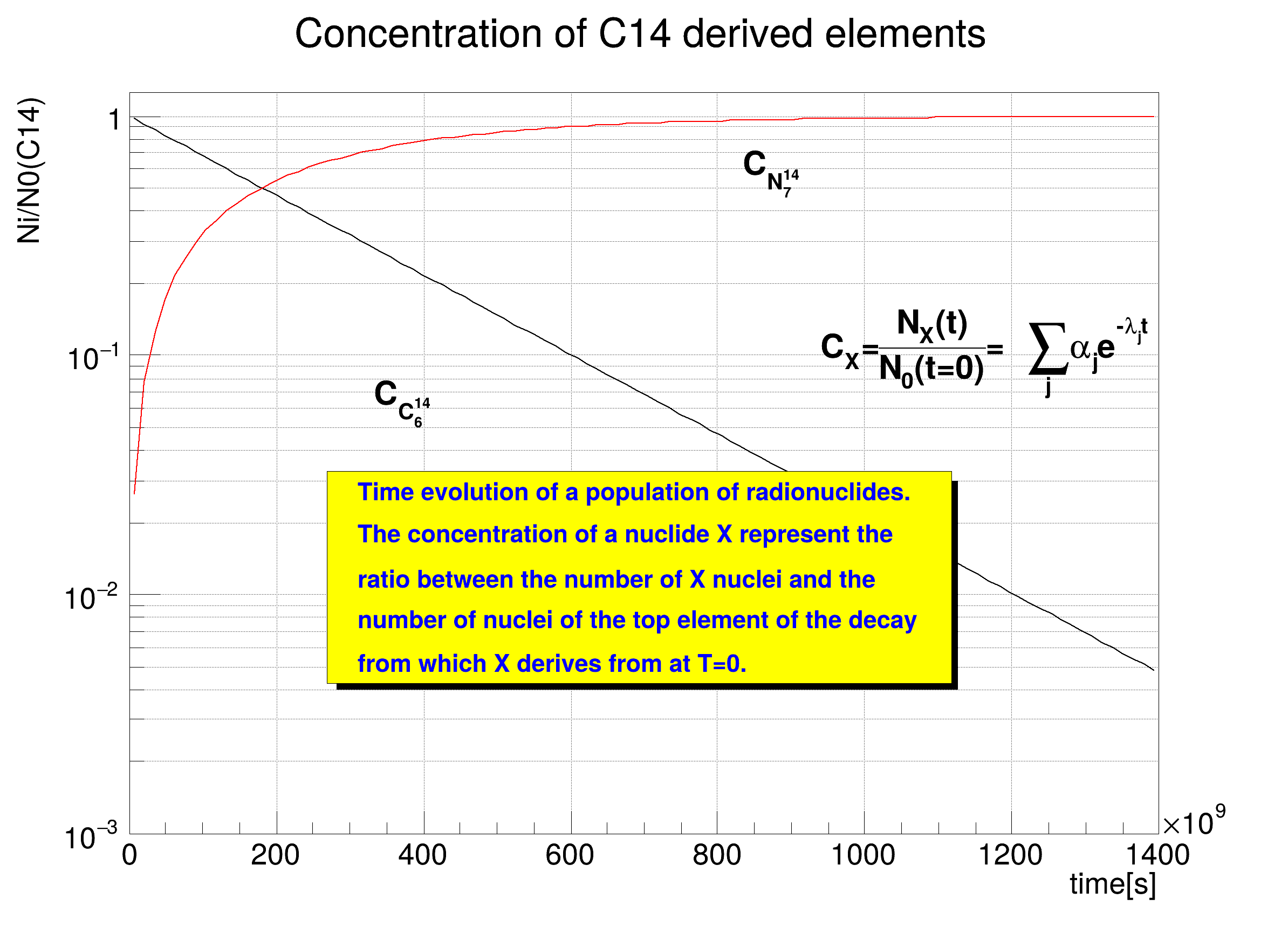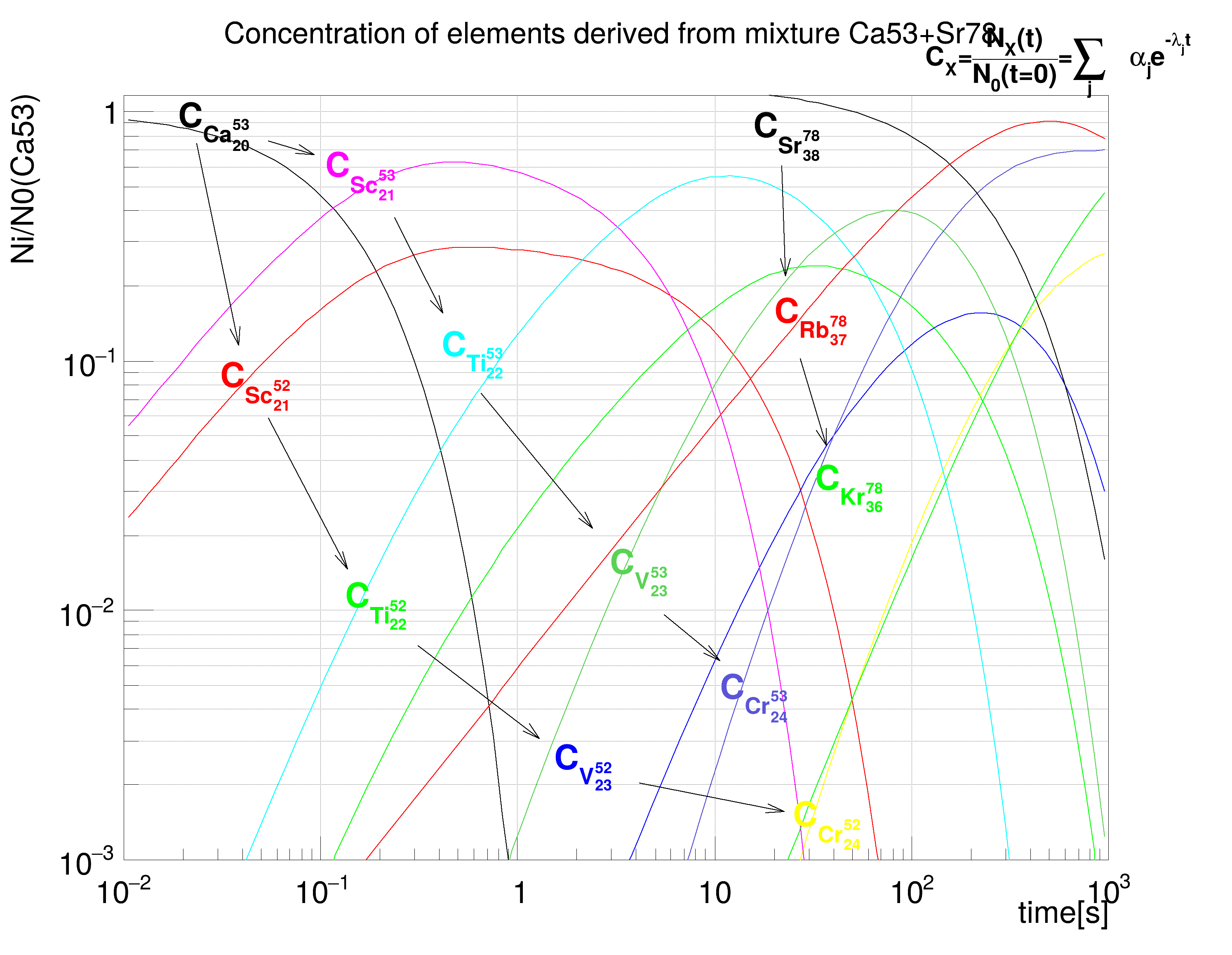ROOT   Reference Guide
Searching...
No Matches

## Detailed Description

Macro that demonstrates usage of radioactive elements/materials/mixtures with TGeo package.

A radionuclide (TGeoElementRN) derives from the class TGeoElement and provides additional information related to its radioactive properties and decay modes.

Int_t atomic_charge,
Int_t isomeric_number)
int Int_t
Definition RtypesCore.h:45
Class representing a radionuclidevoid TGeoManager::SetDefaultRootUnits() { if ( fgDefaultUnits == kRo...
TGeoElementRN * GetElementRN(Int_t ENDFcode) const
Retrieve a radionuclide by ENDF code.

The isomeric number is optional and the default value is 0.

To create a radioactive material based on a radionuclide, one should use the constructor:

TGeoMaterial(const char *name, TGeoElement *elem, Double_t density)
double Double_t
Definition RtypesCore.h:59
char name
Definition TGX11.cxx:110
Base class for chemical elements.
Definition TGeoElement.h:37
Base class describing materials.

To create a radioactive mixture, one can use radionuclides as well as stable elements:

TGeoMixture(const char *name, Int_t nelements, Double_t density);
Mixtures of elements.
void AddElement(Double_t a, Double_t z, Double_t weight)
add an element to the mixture using fraction by weight Check if the element is already defined

Once defined, one can retrieve the time evolution for the radioactive materials/mixtures by using one of the 2 methods:

Double_t precision=0.001)
virtual void FillMaterialEvolution(TObjArray *population, Double_t precision=0.001)
Fills a user array with all the elements deriving from the possible decay of the top element composin...
An array of TObjects.
Definition TObjArray.h:31

To use this method, one has to provide an empty TObjArray object that will be filled with all elements coming from the decay chain of the initial radionuclides contained by the material/mixture. The precision represent the cumulative branching ratio for which decay products are still considered. The POPULATION list may contain stable elements as well as radionuclides, depending on the initial elements. To test if an element is a radionuclide:

bool Bool_t
Definition RtypesCore.h:63
Definition TGeoElement.h:87

All radionuclides in the output population list have attached objects that represent the time evolution of their fraction of nuclei with respect to the top radionuclide in the decay chain. These objects (Bateman solutions) can be retrieved and drawn:

void Draw(Option_t *option="") override
Draw the solution of Bateman equation versus time.
TGeoBatemanSol * Ratio() const

Another method allows to create the evolution of a given radioactive material/mixture at a given moment in time:

virtual TGeoMaterial * DecayMaterial(Double_t time, Double_t precision=0.001)
Create the material representing the decay product of this material at a given time.

The method will create the mixture that result from the decay of a initial material/mixture at TIME, while all resulting elements having a fractional weight less than PRECISION are excluded.void DrawPopulation(TObjArray *vect, TCanvas *can, Double_t tmin=0.,
Double_t tmax=0., Bool_t logx=kFALSE);
{
TGeoManager *geom = new TGeoManager("","");
TGeoElementRN *c14 = table->GetElementRN(14,6);
TGeoElementRN *el1 = table->GetElementRN(53,20);
TGeoElementRN *el2 = table->GetElementRN(78,38);
TGeoMaterial *mat = new TGeoMaterial("C14", c14, 1.3);
printf("___________________________________________________________\n");
mat->Print();
Double_t time = 1.5e11; // seconds
TGeoMaterial *decaymat = mat->DecayMaterial(time);
printf("Radioactive material evolution after %g years:\n", time/3.1536e7);
decaymat->Print();
TGeoMixture *mix = new TGeoMixture("mix", 2, 7.3);
printf("___________________________________________________________\n");
mix->Print();
time = 1000.;
decaymat = mix->DecayMaterial(time);
printf("Radioactive mixture evolution after %g seconds:\n", time);
decaymat->Print();
TObjArray *vect = new TObjArray();
TCanvas *c1 = new TCanvas("c1","C14 decay", 800,600);
c1->SetGrid();
DrawPopulation(vect, c1, 0, 1.4e12);
TLatex *tex = new TLatex(8.35e11,0.564871,"C_{N^{14}_{7}}");
tex->SetTextSize(0.0388601);
tex->SetLineWidth(2);
tex->Draw();
tex = new TLatex(3.33e11,0.0620678,"C_{C^{14}_{6}}");
tex->SetTextSize(0.0388601);
tex->SetLineWidth(2);
tex->Draw();
tex = new TLatex(9.4e11,0.098,"C_{X}=#frac{N_{X}(t)}{N_{0}(t=0)}=\
#sum_{j}#alpha_{j}e^{-#lambda_{j}t}");
tex->SetTextSize(0.0388601);
tex->SetLineWidth(2);
tex->Draw();
TPaveText *pt = new TPaveText(2.6903e+11,0.0042727,1.11791e+12,0.0325138,"br");
pt->AddText("The concentration of a nuclide X represent the ");
pt->AddText("ratio between the number of X nuclei and the ");
pt->AddText("number of nuclei of the top element of the decay");
pt->AddText("from which X derives from at T=0. ");
pt->Draw();
c1->Modified();
vect->Clear();
TCanvas *c2 = new TCanvas("c2","Mixture decay", 1000,800);
c2->SetGrid();
DrawPopulation(vect, c2, 0.01, 1000., kTRUE);
tex = new TLatex(0.019,0.861,"C_{Ca^{53}_{20}}");
tex->SetTextSize(0.0388601);
tex->SetTextColor(1);
tex->Draw();
tex = new TLatex(0.0311,0.078064,"C_{Sc^{52}_{21}}");
tex->SetTextSize(0.0388601);
tex->SetTextColor(2);
tex->Draw();
tex = new TLatex(0.1337,0.010208,"C_{Ti^{52}_{22}}");
tex->SetTextSize(0.0388601);
tex->SetTextColor(3);
tex->Draw();
tex = new TLatex(1.54158,0.00229644,"C_{V^{52}_{23}}");
tex->SetTextSize(0.0388601);
tex->SetTextColor(4);
tex->Draw();
tex = new TLatex(25.0522,0.00135315,"C_{Cr^{52}_{24}}");
tex->SetTextSize(0.0388601);
tex->SetTextColor(5);
tex->Draw();
tex = new TLatex(0.1056,0.5429,"C_{Sc^{53}_{21}}");
tex->SetTextSize(0.0388601);
tex->SetTextColor(6);
tex->Draw();
tex = new TLatex(0.411,0.1044,"C_{Ti^{53}_{22}}");
tex->SetTextSize(0.0388601);
tex->SetTextColor(7);
tex->Draw();
tex = new TLatex(2.93358,0.0139452,"C_{V^{53}_{23}}");
tex->SetTextSize(0.0388601);
tex->SetTextColor(8);
tex->Draw();
tex = new TLatex(10.6235,0.00440327,"C_{Cr^{53}_{24}}");
tex->SetTextSize(0.0388601);
tex->SetTextColor(9);
tex->Draw();
tex = new TLatex(15.6288,0.782976,"C_{Sr^{78}_{38}}");
tex->SetTextSize(0.0388601);
tex->SetTextColor(1);
tex->Draw();
tex = new TLatex(20.2162,0.141779,"C_{Rb^{78}_{37}}");
tex->SetTextSize(0.0388601);
tex->SetTextColor(2);
tex->Draw();
tex = new TLatex(32.4055,0.0302101,"C_{Kr^{78}_{36}}");
tex->SetTextSize(0.0388601);
tex->SetTextColor(3);
tex->Draw();
tex = new TLatex(117.,1.52,"C_{X}=#frac{N_{X}(t)}{N_{0}(t=0)}=#sum_{j}\
#alpha_{j}e^{-#lambda_{j}t}");
tex->SetTextSize(0.03);
tex->SetLineWidth(2);
tex->Draw();
TArrow *arrow = new TArrow(0.0235313,0.74106,0.0385371,0.115648,0.02,">");
arrow->SetFillColor(1);
arrow->SetFillStyle(1001);
arrow->SetLineWidth(2);
arrow->SetAngle(30);
arrow->Draw();
arrow = new TArrow(0.0543138,0.0586338,0.136594,0.0146596,0.02,">");
arrow->SetFillColor(1);
arrow->SetFillStyle(1001);
arrow->SetLineWidth(2);
arrow->SetAngle(30);
arrow->Draw();
arrow = new TArrow(0.31528,0.00722919,1.29852,0.00306079,0.02,">");
arrow->SetFillColor(1);
arrow->SetFillStyle(1001);
arrow->SetLineWidth(2);
arrow->SetAngle(30);
arrow->Draw();
arrow = new TArrow(4.13457,0.00201942,22.5047,0.00155182,0.02,">");
arrow->SetFillColor(1);
arrow->SetFillStyle(1001);
arrow->SetLineWidth(2);
arrow->SetAngle(30);
arrow->Draw();
arrow = new TArrow(0.0543138,0.761893,0.0928479,0.67253,0.02,">");
arrow->SetFillColor(1);
arrow->SetFillStyle(1001);
arrow->SetLineWidth(2);
arrow->SetAngle(30);
arrow->Draw();
arrow = new TArrow(0.238566,0.375717,0.416662,0.154727,0.02,">");
arrow->SetFillColor(1);
arrow->SetFillStyle(1001);
arrow->SetLineWidth(2);
arrow->SetAngle(30);
arrow->Draw();
arrow = new TArrow(0.653714,0.074215,2.41863,0.0213142,0.02,">");
arrow->SetFillColor(1);
arrow->SetFillStyle(1001);
arrow->SetLineWidth(2);
arrow->SetAngle(30);
arrow->Draw();
arrow = new TArrow(5.58256,0.00953882,10.6235,0.00629343,0.02,">");
arrow->SetFillColor(1);
arrow->SetFillStyle(1001);
arrow->SetLineWidth(2);
arrow->SetAngle(30);
arrow->Draw();
arrow = new TArrow(22.0271,0.601935,22.9926,0.218812,0.02,">");
arrow->SetFillColor(1);
arrow->SetFillStyle(1001);
arrow->SetLineWidth(2);
arrow->SetAngle(30);
arrow->Draw();
arrow = new TArrow(27.2962,0.102084,36.8557,0.045686,0.02,">");
arrow->SetFillColor(1);
arrow->SetFillStyle(1001);
arrow->SetLineWidth(2);
arrow->SetAngle(30);
arrow->Draw();
}
void DrawPopulation(TObjArray *vect, TCanvas *can, Double_t tmin,
Double_t tmax, Bool_t logx)
{
Int_t n = vect->GetEntriesFast();
can->SetLogy();
if (logx) can->SetLogx();
for (Int_t i=0; i<n; i++) {
TGeoElement *el = (TGeoElement*)vect->At(i);
TGeoBatemanSol *sol = elem->Ratio();
if (sol) {
sol->SetLineColor(1+(i%9));
sol->SetLineWidth(2);
if (tmax>0.) sol->SetRange(tmin,tmax);
if (i==0) {
sol->Draw();
TF1 *func = (TF1*)can->FindObject(
Form("conc%s",sol->GetElement()->GetName()));
if (func) {
if (!strcmp(can->GetName(),"c1")) func->SetTitle(
"Concentration of C14 derived elements;time[s];Ni/N0(C14)");
else func->SetTitle(
"Concentration of elements derived from mixture Ca53+Sr78;\
time[s];Ni/N0(Ca53)");
}
}
else sol->Draw("SAME");
}
}
}
const Bool_t kFALSE
Definition RtypesCore.h:101
const Bool_t kTRUE
Definition RtypesCore.h:100
R__EXTERN TGeoManager * gGeoManager
char * Form(const char *fmt,...)
Formats a string in a circular formatting buffer.
Definition TString.cxx:2468
Draw all kinds of Arrows.
Definition TArrow.h:29
virtual void SetFillColor(Color_t fcolor)
Set the fill area color.
Definition TAttFill.h:37
virtual void SetLineWidth(Width_t lwidth)
Set the line width.
Definition TAttLine.h:43
virtual void SetLineColor(Color_t lcolor)
Set the line color.
Definition TAttLine.h:40
virtual void SetTextAlign(Short_t align=11)
Set the text alignment.
Definition TAttText.h:42
virtual void SetTextColor(Color_t tcolor=1)
Set the text color.
Definition TAttText.h:44
virtual void SetTextSize(Float_t tsize=1)
Set the text size.
Definition TAttText.h:47
The Canvas class.
Definition TCanvas.h:23
1-Dim function class
Definition TF1.h:213
void SetTitle(const char *title="") override
Set function title if title has the form "fffffff;xxxx;yyyy", it is assumed that the function title i...
Definition TF1.cxx:3549
void SetRange(Double_t tmin=0., Double_t tmax=0.)
TGeoElementRN * GetElement() const
Table of elements.
The manager class for any TGeo geometry.
Definition TGeoManager.h:45
TGeoElementTable * GetElementTable()
Returns material table. Creates it if not existing.
void Print(const Option_t *option="") const override
print characteristics of this material
TGeoMaterial * DecayMaterial(Double_t time, Double_t precision=0.001) override
Create the mixture representing the decay product of this material at a given time.
void FillMaterialEvolution(TObjArray *population, Double_t precision=0.001) override
Fills a user array with all the elements deriving from the possible decay of the top elements composi...
void Print(const Option_t *option="") const override
print characteristics of this material
To draw Mathematical Formula.
Definition TLatex.h:18
const char * GetName() const override
Returns name of object.
Definition TNamed.h:47
Int_t GetEntriesFast() const
Definition TObjArray.h:58
void Clear(Option_t *option="") override
Remove all objects from the array.
TObject * At(Int_t idx) const override
Definition TObjArray.h:164
virtual void Draw(Option_t *option="")
Default Draw method for all objects.
Definition TObject.cxx:274
void SetLogy(Int_t value=1) override
Set Lin/Log scale for Y.
TObject * FindObject(const char *name) const override
void SetLogx(Int_t value=1) override
Set Lin/Log scale for X.
const char * GetName() const override
Returns name of object.
A Pave (see TPave) with text, lines or/and boxes inside.
Definition TPaveText.h:21
virtual TText * AddText(Double_t x1, Double_t y1, const char *label)
Add a new Text line to this pavetext at given coordinates.
void Draw(Option_t *option="") override
Draw this pavetext with its current attributes.
TPaveText * pt
return c1
Definition legend1.C:41
const Int_t n
Definition legend1.C:16
return c2
Definition legend2.C:14